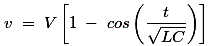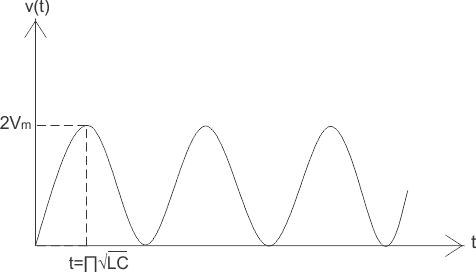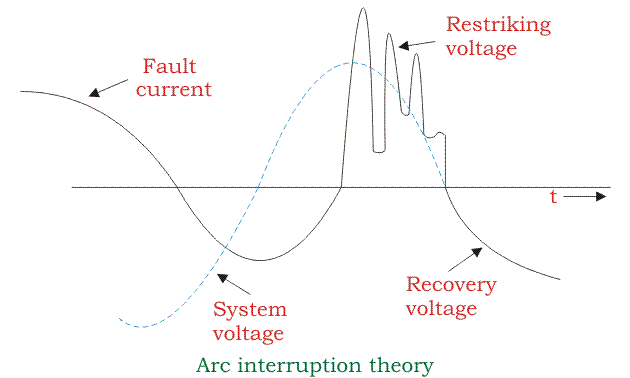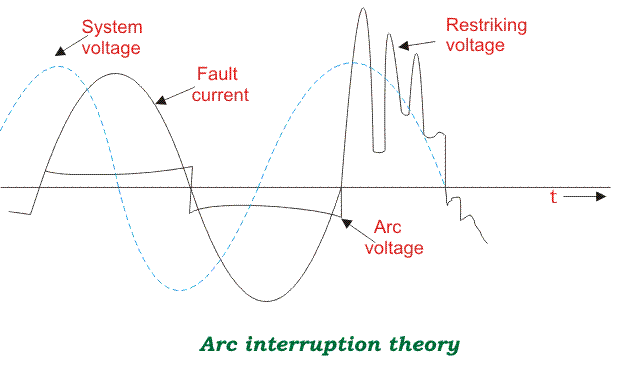# Arc Interruption Theory

The insulating material (may be fluid or air) used in circuit breaker should serve two important functions. They are written as follows:

1. It should provide sufficient insulation between the contacts when circuit breaker opens.
2. It should extinguish the arc occurring between the contacts when circuit breaker opens.

The second point needs more explanation. To understand this point let us consider a situation if there is some fault or short circuit in the system, the relay provides desired signals to the circuit breaker so as to prevent system from ongoing fault. Now when circuit breaker opens its contacts, due to this an arc is drawn. The arc is interrupted by suitable insulator and technique.

## Methods of Arc Interruption

There are two methods by which interruption is done.

1. High resistance method,
2. Low resistance method or current zero interruption method.

In high interruption method we can increase the electrical resistance many times to such a high value that it forces the current to reach to zero and thus restricting the possibility of arc being restruck. Proper steps must be taken in order to ensure that the rate at which the resistance is increased or decreased is not abnormal because it may lead to generation of harmful induced voltages in the system. The arc resistance can be increased by various methods like lengthening or cooling of the arc etc.

## Limitations of High Resistance Method

Arc discharge has a resistive nature due to this most of the energy is received by circuit breaker itself hence proper care should be taken during the manufacturing of circuit breaker like mechanical strength etc. Therefore this method is applied in DC power circuit breaker, low and medium AC power circuit breaker.
Low resistance method is applicable only for ac circuit and it is possible there because of presence of natural zero of current. The arc gets extinguished at the natural zero of the AC wave and is prevented from restricting again by rapid building of dielectric strength of the contact space.

There are two theories which explain the phenomenon of arc extinction:

1. Energy balance theory,
2. Voltage race theory.

Before going in details about these theories, we should know the following terms.

• #### Restriking Voltage

It may be defined as the voltages that appears across the breaking contact at the instant of arc extinction.

• #### Recovery Voltage

It may be defined as the voltage that appears across the breaker contact after the complete removal of transient oscillations and final extinction of arc has resulted in all the poles.

• #### Active Recovery Voltage

It may be defined as the instantaneous recovery voltage at the instant of arc extinction.

• #### Arc Voltage

It may be defined as the voltages that appears across the contact during the arcing period, when the current flow is maintained in the form of an arc. It assumes low value except for the point at which the voltage rise rapidly to a peak value and current reaches to zero.

1. ### Energy Balance Theory

When the contact of circuit breaker are about to open, restriking voltage is zero, hence generated heat would be zero and when the contacts are fully open there is infinite resistance this again make no production of heat. We can conclude from this that the maximum generated heat is lying between these two cases and can be approximated, now this theory is based on the fact that the rate of generation of heat between the the contacts of circuit breaker is lower than the rate at which heat between the contact is dissipated. Thus if it is possible to remove the generated heat by cooling, lengthening and splitting the arc at a high rate the generation, arc can be extinguished.

2. ### Voltage Race Theory

The arc is due to the ionization of the gap between the contact of the circuit breaker. Thus the resistance at the initial stage is very small i.e. when the contact are closed and as the contact separates the resistance starts increasing. If we remove ions at the initial stage either by recombining them into neutral molecules or inserting insulation at a rate faster than the rate of ionization, the arc can be interrupted. The ionization at zero current depends on the voltages known as restriking voltage.

Let us define an expression for restriking voltage. For loss-less or ideal system we have,Here, v = restriking voltage.
V = value of voltage at the instant of interruption.
L and C are series inductor and shunt capacitance up to fault point.
Thus from above equation we can see that lower the value of product of L and C, higher the value of restriking voltage.
The variation of v versus time is plotted below:Now let us consider a practical system, or assume there finite loss in the system. As figure shown below, in this case the restriking voltage is damped out due to the presence of some finite resistance. Here it is assumed that the current lags behind the voltage by an angle (measured in degrees) of 90. However in practical situation angle may varies depending upon time in cycle at which the fault is occurred.Let us consider the effect of arc voltage, if arc voltage is included in the system, there is an increment in the restriking voltage. However this is offset by another effect of an arc voltage which opposes the current flow and making change in the phase of current, thus bringing it more into phase with the applied voltages. Hence the current is not at its peak value when voltage passes through zero value.### Rate of Rise of Restriking Voltage (RRRV)

It is defined as the ratio of peak value of restriking voltage to time taken to reach to peak value. It is one of the most important parameter as if the rate at which the dielectric strength developed between the contacts is greater than RRRV, and then the arc will be extinguishes.

Want To Learn Faster? 🎓
Get electrical articles delivered to your inbox every week.
No credit card required—it’s 100% free.